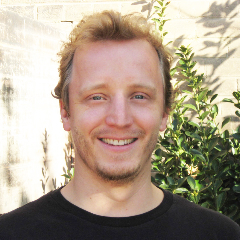Tutor Profile - Ben M.Lives in Bountiful , UT (can travel up to 20 miles)

Bio: I received my Bachelors in physics from the University of Utah and in 2015 completed my PhD in mathematics. I have taught several undergraduate and graduate level courses at the University of Utah, and I have been tutoring since 2005. I greatly enjoy the process of teaching and helping students understand very complicated material. It is fun when they finally get it!

Subjects: Stochastic Processes, Algebra I, Algebra II, AP Calculus AB, AP Calculus BC, Basic Math, Calculus I, Calculus II, Calculus III, Pre-Algebra, Complex Analysis, Differential Equations (ODE), Engineering Calculus I, Engineering Calculus II, Engineering Calculus III, Foundations of Analysis I, IB Higher Level Math, IB Math, Integrated Math 1, Integrated Math 2, Integrated Math 3, Intermediate Algebra, Linear Algebra, Matlab, Numerical Analysis I, Partial Differential Equations (PDE), Pre-Calculus, Quantitative Analysis, Quantitative Reasoning, Secondary Math I (Common Core), Secondary Math II (Common Core), Secondary Math III (Common Core), Stochastic Processes, Trigonometry, Vector Calculus, AP Physics 1, AP Physics 2, Basic Physics, Electricity and Magnetism, General Physics I, General Physics II, High School Physics, IB Physics, Statistical Mechanics, Thermodynamics, Thermodynamics, AP Statistics, Applied Statistics, Basic Statistics, Intro to Inferential Statistics, Intro to Probability, Intro to Statistics

Experience at High Performance Tutoring: Ben has worked at High Performance Tutoring for 4 years and 8 months and has helped 51 of our students learn effectively, build academic confidence, improve grades, and raise test scores. Ben is highly knowledgeable, great at teaching, has a wonderful personality, and has proven over time to be very reliable.